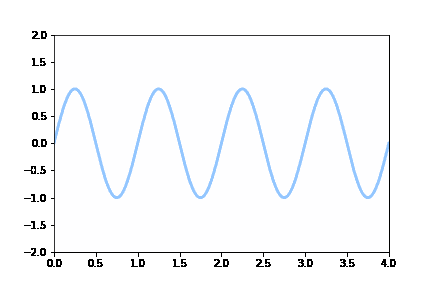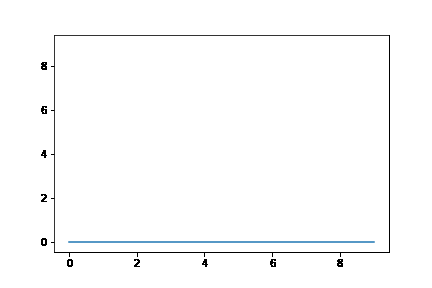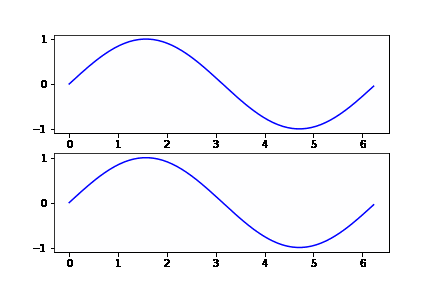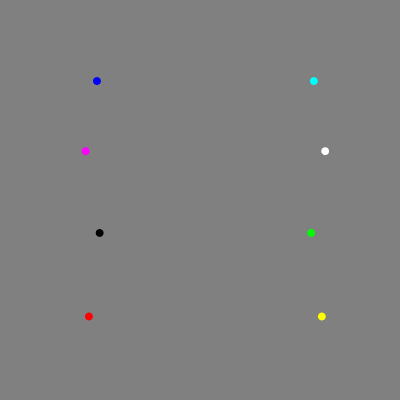# Introduction

In this article, we will learn how we can add animation to the plots by using the matplotlib library. This tutorial will talk about the various examples of matplotlib animations that can be used in your machine learning or data science projects. We’ll also see how these animations can also be saved in a .gif file as well for future reference and usage.

### Importing Matplotlib Library

Before beginning with this matplotlib bar plot tutorial, we’ll need Matplotlib Library. So let’s import Matplotlib

In :
```import matplotlib.pyplot as plt
import numpy as np```

### Example 1:  Matplotlib Animation for Sine Wave

In the 1st example of the matplotlib animation tutorial, we will look at how sine function waves are animated. First, we import FuncAnimation function from the matplotlib animation package.

Now for animating the sine wave function, we need to generate data and then pass the relevant values to the FuncAnimation function.

The result is saved in the form of .gif

In :
```from matplotlib.animation import FuncAnimation
plt.style.use('seaborn-pastel')

fig = plt.figure()
ax = plt.axes(xlim=(0, 4), ylim=(-2, 2))
line, = ax.plot([], [], lw=3)

def init():
line.set_data([], [])
return line,
def animate(i):
x = np.linspace(0, 4, 1000)
y = np.sin(2 * np.pi * (x - 0.01 * i))
line.set_data(x, y)
return line,

anim = FuncAnimation(fig, animate, init_func=init,
frames=200, interval=20, blit=True)

anim.save('sine_wave.gif', writer='imagemagick')
```
Output:### Example 2: Animated Horizontal Line with Matplolib

Here the 2nd example shows how a horizontal line is animated with the help of celluloid library.

With the help of plot function, we are able to plot this animation. Here the animate function of Camera package is used.

In :
```from celluloid import Camera

fig = plt.figure()
camera = Camera(fig)
for i in range(10):
plt.plot([i] * 10)
camera.snap()
animation = camera.animate()
animation.save('celluloid_minimal.gif', writer = 'imagemagick')
```
Output:### Example 3 : Wave Generation in Pair of Plots

This interesting example shows how to plot two animated plots together. For this, we generate data using numpy’s linspace function.

The plot function is called twice for plotting two different wave plots. Again the animate function is used for plotting animated waves.

In :
```from celluloid import Camera

fig, axes = plt.subplots(2)
camera = Camera(fig)
t = np.linspace(0, 2 * np.pi, 128, endpoint=False)
for i in t:
axes.plot(t, np.sin(t + i), color='green')
axes.plot(t, np.sin(t - i), color='green')
camera.snap()

animation = camera.animate()
animation.save('celluloid_subplots.gif', writer = 'imagemagick')
```
Output:### Example 4: Rotating Animation of RGB Cube with Matplotlib

This last example shows you how to animate a 3D Object with the help of the matplotlib library. Again the same libraries and functions are loaded.

With the help of NumPy’s stack function, we are able to get the three axes one over the other. The scatter function helps in plotting the markers in the form of a cube.

In :
```from matplotlib.animation import FuncAnimation
from mpl_toolkits.mplot3d import Axes3D

fig = plt.figure()
ax.set_facecolor((0.5, 0.5, 0.5))

colors=np.stack((X.flatten(),Y.flatten(),Z.flatten()),axis=1)
ax.scatter(X,Y,Z,alpha=1.0,s=50,c=colors,marker='*',linewidth=0)
plt.axis('off')
fig.set_size_inches(5, 5)

def update(i, fig, ax):
ax.view_init(elev=50., azim=i)
return fig, ax

anim = FuncAnimation(fig, update, frames=np.arange(0, 360, 2), repeat=True, fargs=(fig, ax))
anim.save('rgb_cube.gif', dpi=80, writer='imagemagick', fps=24)
```
Output:## Conclusion

We have reached the end of this matplotlib animation tutorial, in this we learned about different types of animations with examples. The plots covered talk about the intricate details that should be taken care of while building a variety of useful animations. We also looked at how animations can be stored into a .gif file for easy usage.

Reference –  Matplotlib Documentation